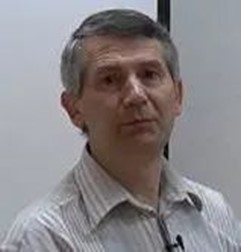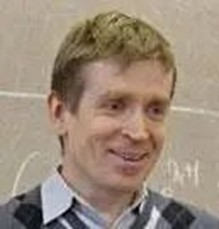01

One-dimensional dynamics

Sergey Kryzhevich

Saint Petersburg State UniversityAbstract: This course is regarded an addition to the regular lecture on Dynamical Systems. Although I deliberately limit myself to one-dimensional dynamics, some principal concepts and proofs of the modern theory of Dynamical systems are introduced. In particular, the course includes

-a brief introduction to Ergodic Theory,

-continuous mappings of a circle,

-continuous and smooth mappings of the segment,

-some facts about the Bifurcation Theory,

-a brief introduction to Interval Exchange Maps,

-an introduction to 1D Complex Dynamics. The syllabus given below is flexible. Some parts of this course can be omitted and provided all the students know them already (this mainly concerns the first part of the course). Meanwhile, the most sophisticated a part of the course (presumably, the Complex Dynamics) can be reduced, if necessary. C. Syllabus, the main content, and the corresponding period distribution (a total of 48 hours, every period of 50 minutes each).

Time：The course starts on February 22, 2023

Wednesdays    15:10-16:00  16:10-17:00

Fridays            18:40-19:30  19:40-20:30

00102941 Periodic and fixed points  (研究生）

00102509 Periodic and Fixed points（本科生）

02

Integrable Systems: the r-matrix Approach

Mikhail Semenov-Tian-Shansky

PDMIAbstract: The present lecture proposal is based on the lectures which the author was giving at the Universite de Bourgogne, Dijon, France during two special semesters on Integrable Systems. Their first version was included in the Russian monograph “Integrable Systems. Group-Theoretical Aspects” published by the author together with A. Reyman in 2003. The notion of classical r-matrix introduced in the late 1970s by Sklyanin has proved to be an extremely useful tool that allows understanding of geometric and algebraic aspects of the Theory of Integrable Systems from a single point of view. The r-matrix approach allows us to take into account naturally several important features which are typical for various concrete examples of Integrable Systems

1.Preliminaries: Poisson Brackets, Poisson and Symplectic Manifolds, Symplectic Leaves.

2.The r-Matrix Method and the Main Theorem

3.Classical r-matrices Related to A-ne Lie Algebras

4.Poisson Lie Groups

5.Duality theory for Poisson Lie groups

6.Lax equations on Lie groups

7.Zero Curvature Equations and Current Algebras

8.Virasoro Algebra and Schroedinger Operators on the Circle

Time：The course starts on February 21, 2023

Tuesdays             18:40-19:30  19:40-20:30

Thursdays            15:10-16:00  16:10-17:00

00102783 The Theory of Integrable Systems         （研究生)

00102399 Random matrices and related topics II    (本科生)

03

Complex-analytic aspects of soliton theory

Domrin Andrei Victorovich

Moscow State UniversityAbstract: The course is intended as an introduction to the theory of(1+1)-dimensional continuous-time integrable systems (soliton equations) from the point of view of complex analysis, with special emphasis on the construction and description of analytic properties of all solutions that are holomorphic concerning the spatial variable \$x\$ and the temporal variable \$t\$ in the neighborhood of a given point. This class of solutions contains multi-soliton solutions, finite-gap solutions, and many others. We define soliton equations of parabolic type as reductions of zero curvature conditions whose coefficients are polynomials in the spectral parameter, describe this class of equations in detail (it contains the famous Korteweg--de Vries equation, nonlinear Schr\"odinger equation, and others), and give a construction of some local holomorphic solutions in terms of the Riemann problem. Then we extend this construction in such a way that it covers all local holomorphic solutions. This may be regarded as the direct and inverse scattering transform for local holomorphic potentials (it is equivalent to the ordinary one whenever both approaches are applicable). It turns out that the resulting scattering data enable us to give a criterion for the solvability of the Cauchy problem for any given flow in the hierarchy as well as a sufficient condition for the extension to a globally meromorphic monodromy-free function of the spatial variable. In particular, solion equations of parabolic type possess a strong form of the Painlev\'e property: every local holomorphic solution extends to a globally meromorphic monodromy-free function of the spatial variable. These and many related results will be given with full proofs (the required background is restricted to the standard courses of complex and functional analysis).

Some results will be stated without proof (for motivation and orientation) and several unsolved problems and conjectures will be highlighted.

Time：The course starts on February 23, 2023

Thursdays            13:00-13:50   14:00-14:50

Fridays                 15:10-16:00  16:10-17:00

00102942 Introduction to contact geometry and nonlinear equations（研究生)

00102510 Introduction to contact geometry and nonlinear differential equations（本科生)

04

Spherical varieties

Timashev Dmitry Andreevich

Moscow State UniversityAbstract: Spherical homogeneous spaces are both classical and modern objects of study in algebra and geometry. Particular examples were studied by geometers since the XIX-th century, starting from spheres and projective spaces and passing to Grassmannians, flag varieties, spaces of quadrics, and, more generally, symmetric spaces. However, the unity of properties and approaches to the study of spherical spaces was well understood not too long ago, which led to an active development of the theory during the last 40 years. Spherical homogeneous spaces lie at the crossroads of algebraic geometry, the theory of algebraic groups, enumerative geometry, harmonic analysis, and representation theory. We shall consider various properties of spherical spaces from viewpoints of algebraic transformation groups, harmonic analysis, and equivariant symplectic geometry.

By standard reasons of algebraic geometry, to solve various problems on a spherical homogeneous space it is helpful to compactify it keeping track of the group action, i.e., to consider equivariant completions or, more generally, open embeddings of a given homogeneous space, called spherical varieties. It is an interesting class of rational algebraic varieties including toric varieties as a special case. Alike the toric case, the classification, and study of spherical varieties rely on certain data of combinatorial nature from discrete and convex geometry: lattices, valuation cones, and colors, colored cones and fans, piecewise linear functions and polytopes, etc.

We shall develop this theory and pass it to applications, which include: the theory of divisors and line bundles on spherical varieties, cellular decomposition, and cohomology, solving enumerative problems on spherical homogeneous spaces, problems in representation theory such as tensor product decompositions, algebraic semigroups, etc. An important property of spherical varieties is Frobenius splitting after reduction to positive characteristic, which has important consequences such as rationality of singularities and vanishing of higher cohomology of nef line bundles. If time allows, we shall discuss Frobenius splitting and also the recent classification of spherical homogeneous spaces based on the concepts of a wonderful variety and a spherical system.

The prerequisites for this course are basic knowledge of algebraic geometry, algebraic groups, and their representations. We shall recall more advanced concepts in the course of the exposition.

Time：The course starts on February 20, 2023

Mondays             15:10-16:00  16:10-17:00

Wednesdays        18:40-19:30  19:40-20:30

00110390 Differential Geometry of Fiber Bundles（研究生)

00137230 Topics on arithmetic geometry            （本科生)

05

Deformation theory

Christopher Brav

Higher School of EconomicsAbstract: Deformation theory in the most general sense the study of how some of the solutions to a system of equations can be perturbed. Typical applications in algebra and geometry include studying perturbations of rings, of varieties, of bundles on varieties, and so on. The general procedure for studying such perturbations is to work innitesimally, order by order in formal parameters, and to consider the obstructions for extending a perturbation to the next higher order. This procedure allows deformations of a given order to be parametrized by linear data (in terms of the ‘tangent complex’ of the problem), while obstructions are encoded in a certain homotopy Lie algebra structure on the shifted tangent complex. Making these ideas precise and fully general requires the introduction of derived algebraic geometry, a generalization of algebraic geometry in which commutative rings are replaced with some kind of derived or homotopical commutative rings (differential graded, simplicial, or E∞, depending on the context). We shall begin the course with an introduction to derived algebraic geometry, in particular the construction of the (co)tangent complex on derived schemes and more general spaces, before formulating and outlining the proof of the main theorem of derived deformation theory that makes precise the sense in which ‘deformations are controlled by differential graded Lie algebras’. Examples that we shall study include deformations of schemes (controlled by the Lie algebra of derived vector elds), deformations of bundles on schemes (controlled by the Lie algebra of derived endomorphisms of the bundle), and deformations of associative algebras (controlled by the Lie algebra of derived derivations). If time permits, we shall discuss more advanced examples arising in non-commutative geometry, such as the Gerstenhaber bracket on Hochschild cochains controlling deformations of a differential graded category, and the deformation theoretic proof of Hochschild-Kostant-Rosenberg theorem identifying Hochschild homology of a scheme with shifted Dolbeault cohomology, via functions on the derived loop space.

Time：The course starts on February 20, 2023

Mondays              18:40-19:30  19:40-20:30

Thursdays            18:40-19:30  19:40-20:30

00103109 Triangulated and Derived Categories in Algebra and Geometry（研究生)

00132110 Topics on Core Mathematic Subjects I（本科生)